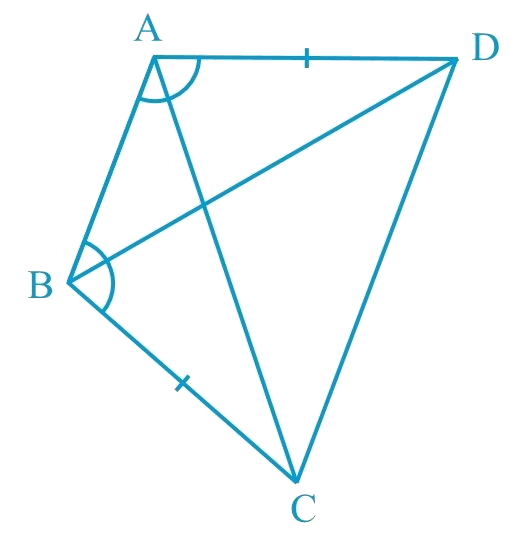# Ex.7.1 Q2 Triangles Solution - NCERT Maths Class 9

Go back to  'Ex.7.1'

## Question

$$ABCD$$ is a quadrilateral in which $$AD = BC$$ and $$\angle DAB = \angle CBA$$ (See the given figure).

Prove that

(i) $$\Delta ABD \cong \Delta BAC$$

(ii) $$BD = AC$$

(iii) $$\Delta ABD=\Delta BAC$$

Video Solution
Triangles
Ex 7.1 | Question 2

## Text Solution

What is Known?

${AD}={BC}\ \text{and}\ \angle {DAB}=\angle {CBA}$

To prove:

(i) $$\Delta {{ABD}} \cong \Delta {{BAC}}$$

(ii) $${{BD}} = {{AC}}$$

(iii) $$\angle {{ABD}} = \angle {{BAC}}$$

Reasoning:

We can show two sides and included of are equals to corresponding sides and included angle of by using $$\rm{SAS}$$ congruency criterion both triangles will be congruent. Then we can say corresponding parts of congruent triangle will be equal.Steps:

In $$\Delta ABD$$ and $$\Delta BAC$$,

\begin{align} AD &= BC \;\text{(Given)}\\\Delta DAB &=\Delta CBA \;\text{(Given)}\\AB &= BA \;\text{(Common)}\\\therefore \Delta ABD &\cong \Delta BAC\\& \text{(By congruence}\,\text{rule})\\\\\end{align}

\begin{align}\therefore BD &= AC \;\text{(By CPCT )}\\& \text{and}\\\angle ABD &=\angle BAC \;\text{(By CPCT )}\end{align}

Video Solution
Triangles
Ex 7.1 | Question 2

Learn from the best math teachers and top your exams

• Live one on one classroom and doubt clearing
• Practice worksheets in and after class for conceptual clarity
• Personalized curriculum to keep up with school